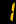# IGCSE

IGCSE Mathematics encourages the development of mathematical knowledge as a key life skill, and as a basis for more advanced study. Its aims to build learners' confidence by helping them develop a feel for numbers, patterns and relationships, and places a strong emphasis on solving problems and presenting and interpreting results. Learners also gain an understanding of how to communicate and reason using mathematical concepts.

### Topics will be taught:

1. Number
2. Algebra and graphs
3. Geometry
4. Mensuration
5. Co-ordinate geometry
6. Trigonometry
7. Matrices and transformations
8. Probability
9. Statistics

### Learning Objectives

• develop their mathematical knowledge and oral, written and practical skills in a way which encourages confidence and provides satisfaction and enjoyment
• read mathematics, and write and talk about the subject in a variety of ways
• develop a feel for number, carry out calculations and understand the significance of the results obtained
• apply mathematics in everyday situations and develop an understanding of the part which mathematics plays in the world around them
• solve problems, present the solutions clearly, check and interpret the results
• develop an understanding of mathematical principles
• recognise when and how a situation may be represented mathematically, identify and interpret relevant factors and, where necessary, select an appropriate mathematical method to solve the problem
• use mathematics as a means of communication with emphasis on the use of clear expression
• develop an ability to apply mathematics in other subjects, particularly science and technology
• develop the abilities to reason logically, to classify, to generalise and to prove 11. appreciate patterns and relationships in mathematics
• produce and appreciate imaginative and creative work arising from mathematical ideas
• develop their mathematical abilities by considering problems and conducting individual and co-operative enquiry and experiment, including extended pieces of work of a practical and investigative kind
• appreciate the interdependence of different branches of mathematics
• acquire a foundation appropriate to their further study of mathematics and of other disciplines